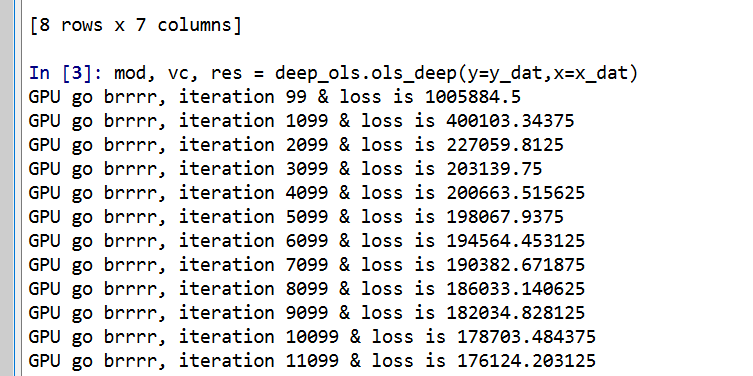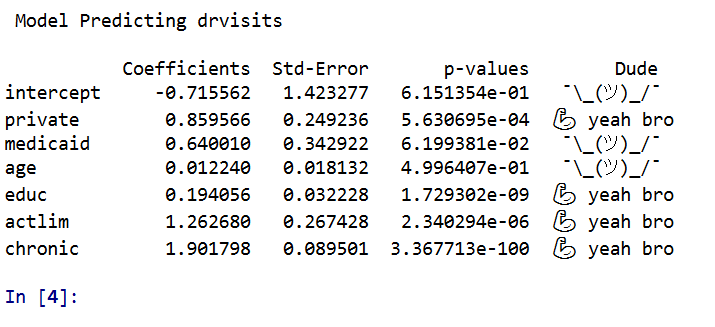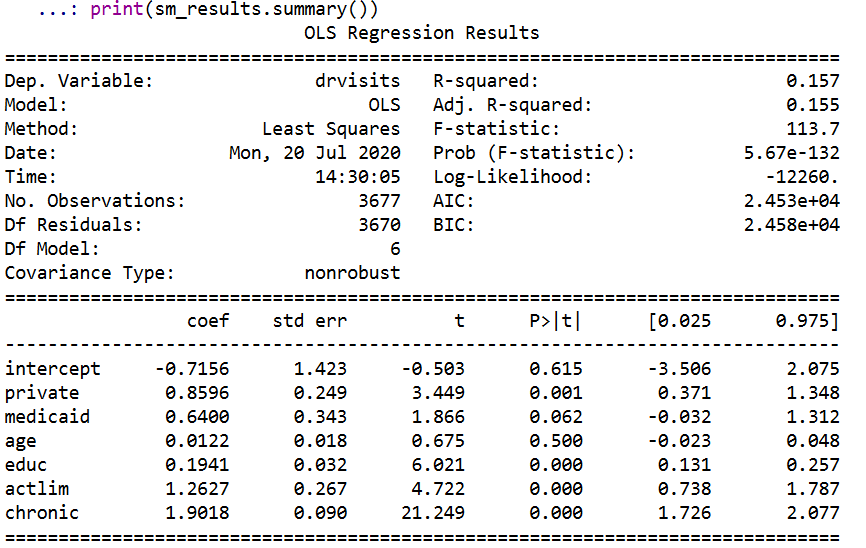# GPU go brrr: Estimating OLS (with standard errors) via deep learning

So a bunch of my criminologists friends have methods envy. So to help them out, I made some python functions to estimate OLS models using pytorch (a deep learning python library). So if you use my functions, you can just append something like an analysis with GPU accelerated deep learning to the title of your paper and you are good to go. So for example, if your paper is `An analysis of the effects of self control on asking non-questions at an ASC conference`, you can simply change it to `A GPU accelerated deep learning analysis of the effects of self control on asking non-questions at an ASC conference`. See how that works, instantly better.

Code and data saved here. There are quite a few tutorials on doing OLS in deep learning libraries, only thing special here is I also calculate the standard errors for OLS in the code as well.

# python code walkthrough

So first I just import the libraries I need. Then change the directory to wherever you saved the code on your local machine, and then import my `deep_ols` script. The `deep_ols` script also relies on the `scipy.stats` library, but besides pytorch it is the usual scientific python stack.

``````import os
import sys
import torch
import statsmodels.api as sm
import pandas as pd
import numpy as np

###########################################
#Setting the directory and importing
#My deep learning OLS function

my_dir = r'C:\Users\andre\OneDrive\Desktop\DeepLearning_OLS'
os.chdir(my_dir)
sys.path.append(my_dir)
import deep_ols
############################################``````

For the dataset I am using, it is a set of the number of doctor visits I took from some of the Stata docs.

``````#Data from Stata, https://www.stata-press.com/data/r16/gsem_mixture.dta
#see pg 501 https://www.stata.com/manuals/sem.pdf

visit_dat['intercept'] = 1
y_dat = visit_dat['drvisits']
x_dat = visit_dat[['intercept','private','medicaid','age','educ','actlim','chronic']]

print( y_dat.describe() )
print( x_dat.describe() )``````

Then to estimate the model it is simply below, the function returns the torch model object, the variance/covariance matrix of the coefficients (as a torch tensor), and then a nice pandas data frame of the results.

``mod, vc, res = deep_ols.ols_deep(y=y_dat,x=x_dat)``As you can see, it prints out GPU accelerated loss results so fast for your pleasure.

Then, like a champ you can see the OLS results. P-values < 0.05 get a strong arm, those greater than this get a shruggie. No betas to be found in my model results.Just to confirm, I show you get the same results using `statsmodel`.

``````stats_mod = sm.OLS(y_dat,x_dat)
sm_results = stats_mod.fit()
print(sm_results.summary())``````So get on my level bro and start estimating your OLS models with a GPU.

# Some Notes

First, don’t actually use this in real life. I don’t do any pre-processing of the data, so if you have some data that is not on a reasonable scale (e.g. if you had a variable income going from 0 to \$500,000) all sorts of bad things can happen. Second, it is not really accelerated – I mean it is on the GPU and the GPU goes brr, but I’m pretty sure this will not ever be faster than typical OLS libraries. Third, this isn’t really “deep learning” – I don’t have any latent layers in the model.

Also note the standard errors for the coefficients are just calculated using a closed form solution that I pilfered from this reddit post. (If anyone has a textbook reference for that would appreciate it! I Maria Kondo’d most of my text books when I moved out of my UTD office.) If I figure out the right gradient calculations, I could probably also add ‘robust’ somewhere to my suggested title (by using the outer product gradient approach to get a robust variance/covariance matrix for the coefficients).

As a bonus in the data file, I have a python script that shows how layers in a vanilla deep learning model (with two layers) are synonymous with a particular partial least squares model. The ‘relu activation function’ is equivalent to a constraint that restricts the coefficients to be positive, but otherwise will produce equivalent predictions.

You could probably do some nonsense of saving the Jacobian matrix to get standard errors for the latent layers in the neural network if you really wanted to.

To end, for those who are really interested in deep learning models, I think a better analogy is that they are just a way to specify and estimate a set of simultaneous equations. Understanding the nature of those equations will help you relate how deep learning is similar to regression equations you are more familiar with. The neuron analogy is just nonsense IMO and should be retired.